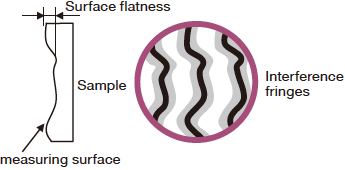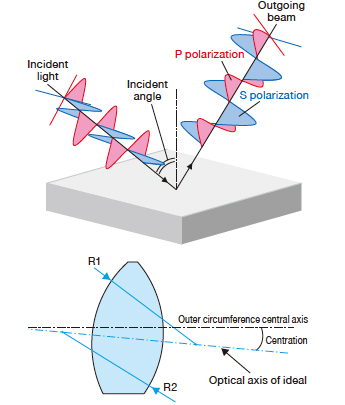# Optics & Optical Coatings

## Guide

### Explanation of Basic Terms

#### Surface Accuracy

A value indicating the deviation between the polished surface and the ideal flat surface.It is also called reflected wavefront distortion because it is measured using an interferometer which measures wavefronts.Surface accuracy is expressed in units of [λ], which uses the number of interference fringes.λ indicates the wavelength 632.8nm of a He-Ne laser used in interferometers.In addition, there are two notations used for surface accuracy, PV and RMS.
PV stands for Peak to Valley (difference between the maximum value and the minimum value),and RMS stands for Root Mean Square (least mean square).Empirically, the RMS value is about 1/3 of the PV value.The PV is often used for simple shapes such as a flat surface, and the surface accuracy shown in this catalog uses the PV value.
For example, the surface accuracy of PV λ/2 indicates that the deviation is maximum 316.5nm from the ideal flat surface.#### Surface Quality (Scratch-Dig)

The standard of scratch on processed optics or coated optics is appeared as; long mark for scratch and deep mark for dig.
If the scratch-dig is 20-10, there are no scratches wider than 2µm, and no digs larger than 100µm.
SigmaKoki adopts the MIL-PRF-13830B standard, which consist of visual comparaison inspection of criteria sample.

#### Laser Damage Threshold

TWhen you irradiate an optic with a high energy pulse laser, it may cause damage to the coating or glass material of the optic.
Laser damage threshold is the energy density [J/cm2] of laser light that will start causing damage to the optic.
Sigma Koki employs the S-on-1 test specified by the international standard ISO21254 for the laser damage threshold.
By comparing the laser damage threshold of optics with the energy density*4 of the laser to be used, you can select the optics that can withstand the laser.
Note that laser damage threshold is not an issue for pulse laser (10ns pulse width) of 100mJ/cm2 or lower, or CW laser of 0.5W or lower.

*4 Energy density [J/cm2] = Laser energy [J] / (π × Beam radius [cm]2)

#### P and S Polarization

When light enters a glass surface at an angle, the reflectance changes according to the polarization orientation of the incident light.
P polarization is light waves oscillating in-plane including those of the normal vector to glass surface and incident beam, while S polarization is light waves oscillating orthogonal to the P polarization. Polarization states other than these can be considered combinations of P and S polarization in varied distribution ratio.
The reflectance of P and S polarization is determined by incident angle and the refractive index of glass, but since each of them follows a different law, the reflectance of P polarization is different from that of S polarization.

#### Angular Deviation of Beam

When an optic is inserted in an optical path of a laser beam, the beam sometimes tilts.
The angle of the tilt from the original beam is called angular deviation of beam.
Angular deviation of beam is determined by the parallelism and refractive index, and in BK7, the parallelism of 1 minute is equivalent to the angular deviation of beam of about 0.5 minutes.#### Centration

When a lens rotates with reference to the outer diameter of the lens, if the lens is ideal, the transmitted beam or focused beam will not move when the lens rotates, but if there is deviation between the central axis of the outer diameter of the lens deviates and the optical axis of the ideal lens, the beam moves as if drawing a circle.
The angular deviation between the transmitted beam and the rotation axis is called centration.

#### Reflectance and Transmittance Wavelength Characteristics Data

The catalog shows a graph of reflectance or transmittance wavelength characteristics for most of the optics listed.

This graph of reflectance or transmittance wavelength characteristics is measured using a spectrophotometer. *5 The catalog shows a wide wavelength range in addition to the range guaranteed by design, but because the values out of the guaranteed range have poor reproducibility and significantly vary among each optic, it is dangerous to trust them.
For a beamsplitter or the like, only the graph of transmittance is listed without the graph of reflectance. The reflectance is however easily deduced by inverting the vertical axis of the graph because no absorption occurs in the case of dielectric multi-layer coating.*6 In addition, incident angle and polarization orientation are specified for measured data. For measurement of reflectance, the incident angle is 5 degrees or 45 degrees, and for transmittance, it is 0 degree or 45 degrees.
Reflection type optics of 45 degrees incidence are listed with a graph of P and S polarization. This is because, in dielectric multi-layer coating, the reflectance characteristics significantly changes depending on the polarization orientation. If the catalog cannot show a graph of P and S polarization due to a space constraint, then it shows the mean value of S and P polarization.

*5 Simulation data of thin film design is used for some optics.
*6 In case of chromium coatings, 1 - Transmittance = Reflectance is not true due to absorption.